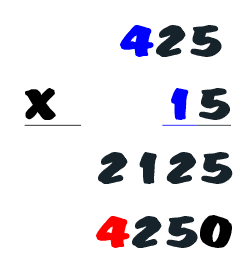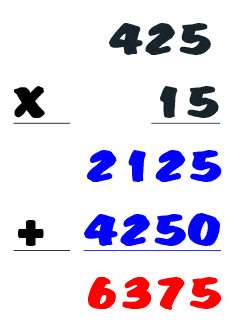The K5 Learning Blog urges parents to be pro-active in helping their children reach their full academic potential.

K5 Learning
provides free worksheets, workbooks and an online reading and math program for kindergarten to grade 5 students.# 2 Easy Ways to do Long Multiplication

Long multiplication is intimidating, especially when you multiply large numbers. Here are two easy ways to do long multiplication.

## Method 1: the shortcut method

Let’s say you are multiplying 425 by 15.

Write down one number next to the other.Split the smaller number into tens and ones. In this case that would be 10 and 5.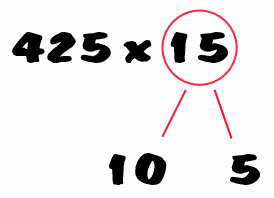Multiply the large number by number in the tens. In this case that’s the 10.Multiply the large number by the number in the ones. In this case that’s the 5. You can even break that down – 400 x 5 = 2,000 and 25 x 5 = 125. Adding 2,000 and 125 equals 2,125.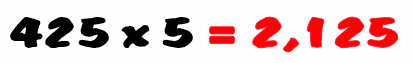Add the two sums: 4,250 and 2,125. You can do this the traditional way.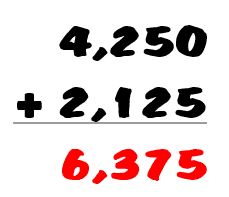## Method 2: standard long multiplication

Write down the large number above the smaller number. Make sure they are aligned so the ones line up, the tens line up and the hundred sits to the left.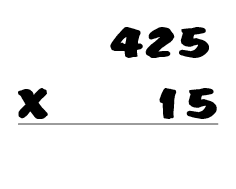Multiply the number in the ones place of the bottom number with the number in the ones place of the top number.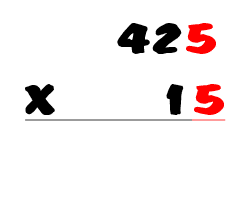5 x 5 is 25. Write the ones digit under the line. In this case it’s 5. And you will be carrying the 2. Write the 2 over the tens pace number. In this case that will be the 2.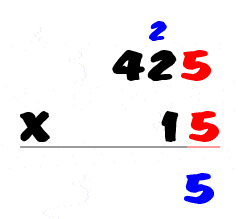Multiply the number in the ones place of the bottom number by the number in the tens place of the top number. In this case that’s 5 x 2, which equals 10. Add the 2 carried over, so that makes the sum 12. Write a 2 next to the five and carry the 1 to above the hundreds number, which is 4.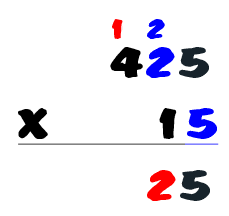Multiply the number in the ones place of the bottom number by the number in the hundreds place of the top number. So, multiply 5 by 4. And add the 1 you carried, making the sum 21.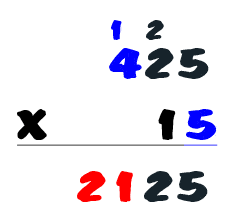Now it’s time to move to the tens column. First, write a zero in the ones column.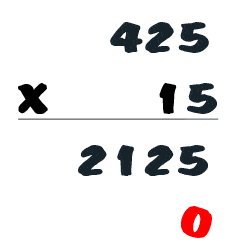Multiply the number in the tens place of the bottom number by the number in ones place of the top number. So multiply the 1 by the 5, which makes 5.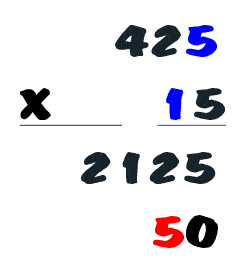Multiply the number in the tens place of the bottom number by the number in tens place of the top number. Multiply 1 by 2, which equals 2.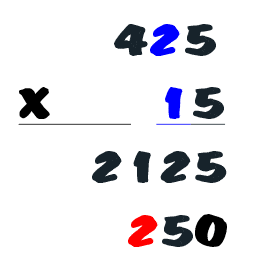Multiply the number in the tens place of the bottom number by the number in hundreds place of the top number. Multiply 1 by 4, which equals 4.